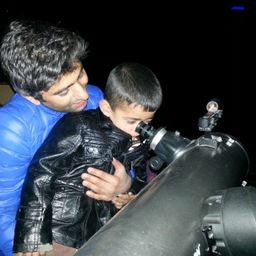Gravitation

### Discussion

You must be signed in to discuss.
##### Top Physics 101 Mechanics Educators##### Andy C.

University of Michigan - Ann Arbor##### Aspen F.

University of Sheffield##### Jared E.

University of Winnipeg

Lectures

Join Bootcamp

### Video Transcript

we know the masa proton muscle for Dani cools 1.67 multiplied by 10 to the power minus 27 kilogram. Uh, party. Let's calculate rest energy. So rest Energy is equal to M c square M C square. And here I am is 1.67 Multiply by 10 to the power minus 27 kilogram multiplier e speed of light, which is tree multiply by 10 to the power it We took her second and we have square and this equals mine. 130 Mine my number in party nine Mega electron volt. So this is a call to 939. Let me write tongues cooling. Well, uh, this is mine 100 attorney nine Mega Electron walked. Let's do part B in Barbie. Yeah, will calculate the total energy. Well, he is equal to resting duty divided by ah screen Rudolph one minus we squared, divided by C Square. Let's plug in the values Well rest Energies Mine 129. Mega Leckrone world Dubai, Dubai Ah, screen route off one minus Well, we is given, which is, um, 0.950 times the speed of light see. Script gets cancelled. See? Scaring. We have just 2.950 with square and simplifying this we get Ah, 3010 Mega electron. Walt Mega Electron walked and you park Si We calculate a canopic energy. Well, kinetic energy equals our difference off e and rest in receipt. Well, is trees either 10 and rest. Energies. Mind 130. Mine. Mega Electron, Walt. Mmm. Inarticulate musical to £2 market and 70 Mega Electron won't.#### Topics

Gravitation

##### Top Physics 101 Mechanics Educators##### Andy C.

University of Michigan - Ann Arbor##### Aspen F.

University of Sheffield##### Jared E.

University of Winnipeg

Lectures

Join Bootcamp